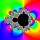Piano

If Suzan practicing 10 minutes at Monday; every other day she wants to practice 2 times as much as the previous day, how many hours and minutes will have to practice on Friday?

Result

x = 2:40 (hh:mm)Solution:Leave us a comment of example and its solution (i.e. if it is still somewhat unclear...):Be the first to comment!Next similar examples:

1. Geometric progression 2There is geometric sequence with a1=5.7 and quotient q=-2.5. Calculate a17.
2. Six termsFind the first six terms of the sequence a1 = -3, an = 2 * an-1
3. Theorem proveWe want to prove the sentence: If the natural number n is divisible by six, then n is divisible by three. From what assumption we started?
4. Geometric progressionFill 4 numbers between 4 and -12500 to form geometric progression.
5. CoefficientDetermine the coefficient of this sequence: 7.2; 2.4; 0.8
6. Three workshopsThere are 2743 people working in three workshops. In the second workshop works 140 people more than in the first and in third works 4.2 times more than the second one. How many people work in each workshop?
7. GP membersThe geometric sequence has 10 members. The last two members are 2 and -1. Which member is -1/16?
8. SequenceWrite the first 6 members of these sequence: a1 = 5 a2 = 7 an+2 = an+1 +2 an
9. LegsCancer has 5 pairs of legs. The insect has 6 legs. 60 animals have a total of 500 legs. How much more are cancers than insects?
10. LineIt is true that the lines that do not intersect are parallel?
11. PIN - codesHow many five-digit PIN - code can we create using the even numbers?
12. AverageIf the average(arithmetic mean) of three numbers x,y,z is 50. What is the average of there numbers (3x +10), (3y +10), (3z+10) ?
13. Geometric sequence 4It is given geometric sequence a3 = 7 and a12 = 3. Calculate s23 (= sum of the first 23 members of the sequence).
14. BlocksThere are 9 interactive basic building blocks of an organization. How many two-blocks combinations are there?
15. AP - simpleDetermine the first nine elements of sequence if a10 = -1 and d = 4
16. Geometric progression 48,4√2,4,2√2
17. Reference angleFind the reference angle of each angle: